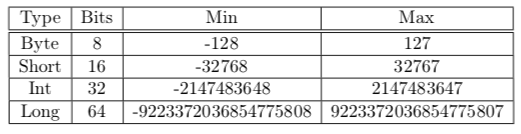# Simple binary numbers conversion

Consider the following

``````scala> val a : Int = 1729
val a: Int = 1729

scala> val b : Int = Integer.parseInt(a.toBinaryString, 2)
val b: Int = 1729
``````

Now take a bigger number that to the best of my knowledge still belong to the range of `Int` according to this table (from Introduction to Programming and Problem-Solving Using Scala, 2nd Edition, by Mark C. Lewis and Lisa L. Lacher, 2016)``````scala> val x : Int = -2147483648
val x: Int = -2147483648

scala>  val y : Int = Integer.parseInt(x.toBinaryString, 2)
java.lang.NumberFormatException: For input string: "10000000000000000000000000000000"
at java.lang.NumberFormatException.forInputString(NumberFormatException.java:65)
at java.lang.Integer.parseInt(Integer.java:583)
... 38 elided

``````

What is the problem? Notice that I am using a popular answer How to write binary literals in Scala? - Stack Overflow

I’m not familiar with `toBinaryString` but it seems to strip the ‘-’ sign.

When I add the sign back manually, it works:

``````val x : Int = -2147483648
Integer.parseInt(s"-\${x.toBinaryString}", 2) // -2147483648: scala.Int
``````

Edit: This is expected – `x.toBinaryString` is the same as `Integer.toBinaryString(x)`, see its javadoc here: Integer (Java SE 11 & JDK 11 )

@cestLaVie Binary numbers do not have minusesHmm, I don’t agree that binary numbers have no minuses. It’s only that the 2s complement representation encodes the sign differently.

To add to the answer: Use `Integer.parseUnsignedInt(s, 2)` to get back the original number in your example:

``````val x : Int = -2147483648
Integer.parseUnsigendInt(x.toBinaryString, 2) // -2147483648: scala.Int

``````